### Home > CALC > Chapter 10 > Lesson 10.1.3 > Problem10-21

10-21.
1. Determine the convergence or divergence of each of the following integrals. Homework Help ✎

1.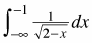2.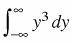3.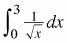4.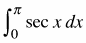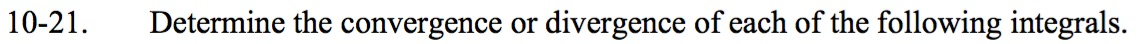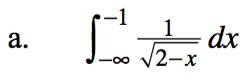$=\lim_{a\to-\infty}\int_{a}^{-1}\frac{1}{\sqrt{2-x}}dx$

$=\left.\lim_{a\to-\infty}\big(2-\sqrt{2-x}\big)\right|_{a}^{-1}$

$\lim_{a\to -\infty}(2\sqrt{3}-2\sqrt{2-a})$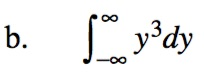$\lim_{b\to -\infty}\int_b^0 y^3dy+\lim_{b\to \infty}\int_0^b y^3dy$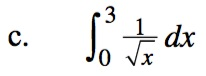$\lim_{c\to 0}\int_c^3\frac{1}{\sqrt{x}}dx$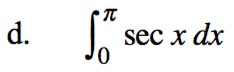sec(x) is undefined at π/2, so this integral will need to be split in two and limits used.

$\int\sec(x)dx=\ln\left|{\sec(x)+\tan(x)}\right|+C$